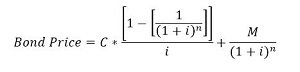# Westover's has an outstanding bond with a coupon rate of 6.5% that matures in 12 years. The bond...

Westover's has an outstanding bond with a coupon rate of 6.5% that matures in 12 years. The bond pays interest semi annually. What is the market price of a $1,000 face value bond if the yield to maturity is 7.13%? ## Bond Price: Bond price is the present value of all expected future cash flows from holding the bond. The cash flows generally expected from holding the bond are the coupons received and the maturity amount received at the end of bond period. ## Answer and Explanation: Bond Price is given as:Here, C is the periodic coupon = 1000 x 6.5% x 1/2 =$32.50

M is the maturity amount = \$1,000.00

i is the periodic...

See full answer below.

Become a Study.com member to unlock this answer! Create your account

#### Learn more about this topic:How to Price Bonds: Formula & Calculation

from Finance 104: Financial Management

Chapter 2 / Lesson 5
2.7K